# Giles Gardam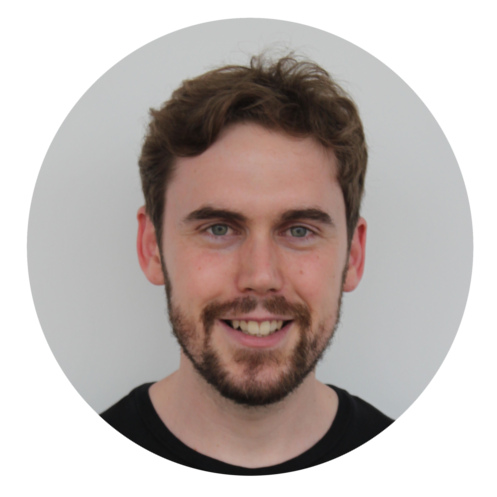I am a postdoc in geometric group theory working in the Topology Group at the WWU Münster (job title: "akademischer Rat a.Z." ~ research associate). My graduate studies were supervised by Martin Bridson in Oxford and afterwards I was a postdoc for a year at the Technion before participating in the Hausdorff Trimester Program Logic and Algorithms in Group Theory in Bonn (September to December 2018). I did my undergraduate studies at the University of Sydney and wrote my honours thesis under the supervision of Anne Thomas.

I successfully defended my DPhil thesis on 1 September 2017.

You can contact me at <first initial><last name>@uni-muenster.de.

## Research interests

I'm interested in obstructions to negative and non-positive curvature (for instance, in what settings are subgroups like Baumslag-Solitar groups the only obstruction?), one-relator groups, group boundaries, finiteness properties, profinite rigidity, minimal presentations (e.g. deficiency), group laws, algorithms and compexity in group theory. My interest in computational group theory is not purely theoretical: I code as appropriate.

## Papers

I have an arXiv page.

### Published or accepted for publication

• Finite groups of arbitrary deficiency, arXiv:1705.02040 journal version
Bull. London Math. Soc. 49 (2017), 1100–1104

The deficiency of a group is the maximum over all presentations for that group of the number of generators minus the number of relators. Every finite group has non-positive deficiency. We show that every non-positive integer is the deficiency of a finite group – in fact, of a finite $$p$$-group for every prime $$p$$. This completes Kotschick's classification of the integers which are deficiencies of fundamental groups of compact Kähler manifolds.• The geometry of one-relator groups satisfying a polynomial isoperimetric inequality with Daniel Woodhouse, arXiv:1711.08755 journal version
Proc. Amer. Math. Soc. 147 (2019), 125–129

For every pair of positive integers $$p > q$$ we construct a one-relator group $$R_{p,q}$$ whose Dehn function is $$\simeq n^\alpha$$ where $$\alpha = 2 \log_2(2p / q)$$. The group $$R_{p,q}$$ has no subgroup isomorphic to a Baumslag-Solitar group $$BS(m,n)$$ with $$m \neq \pm n$$, but is not automatic, not CAT(0), and cannot act freely on a CAT(0) cube complex. This answers a long-standing question on the automaticity of one-relator groups and gives counterexamples to a conjecture of Wise.• Detecting laws in power subgroups, arXiv:1705.09348 journal version
to appear in Comm. Algebra
(Note: arXiv version will be updated after embargo period from journal. Until then please bear with the typesetting of the journal version and semidirect products written $$N, \rtimes, K$$.)

A group law is said to be detectable in power subgroups if, for all coprime $$m$$ and $$n$$, a group $$G$$ satisfies the law if and only if the power subgroups $$G^m$$ and $$G^n$$ both satisfy the law. We prove that for all positive integers $$c$$, nilpotency of class at most $$c$$ is detectable in power subgroups, as is the $$k$$-Engel law for $$k$$ at most 4. In contrast, detectability in power subgroups fails for solvability of given derived length: we construct a finite group $$W$$ such that $$W^2$$ and $$W^3$$ are metabelian but $$W$$ has derived length $$3$$. We analyse the complexity of the detectability of commutativity in power subgroups, in terms of finite presentations that encode a proof of the result.• Profinite rigidity in the SnapPea census, arXiv:1805.02697
to appear in Exp. Math.

A well-known question asks whether any two non-isometric finite volume hyperbolic 3-manifolds are distinguished from each other by the finite quotients of their fundamental groups. At present, this has been proved only when one of the manifolds is a once-punctured torus bundle over the circle. We give substantial computational evidence in support of a positive answer, by showing that no two manifolds in the SnapPea census of 72 942 finite volume hyperbolic 3-manifolds have the same finite quotients.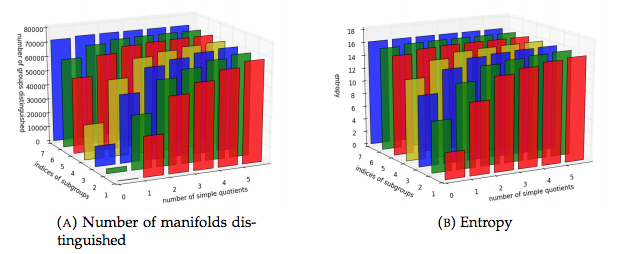### Preprints

• Cannon–Thurston maps for CAT(0) groups with isolated flats, with Benjamin Beeker, Matthew Cordes, Radhika Gupta, and Emily Stark, arXiv:1810.13285

Mahan Mitra (Mj) proved Cannon–Thurston maps exist for normal hyperbolic subgroups of a hyperbolic group. We prove that Cannon–Thurston maps do not exist for infinite torsion-free normal hyperbolic subgroups of a non-hyperbolic CAT(0) group with isolated flats with respect to the visual boundaries. We also show Cannon–Thurston maps do not exist for infinite torsion-free infinite-index normal CAT(0) subgroups with isolated flats in a non-hyperbolic CAT(0) group with isolated flats. We determine the isomorphism types of the normal subgroups in the above two settings.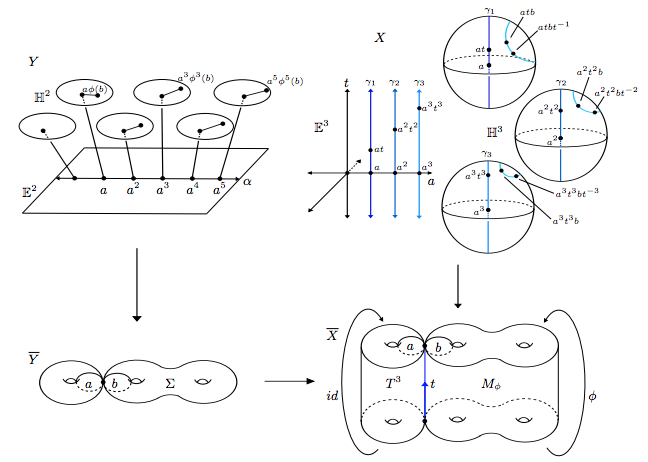• Minimal sizeable graphs, arXiv:1808.09505 appendix to the paper Hyperbolic Groups with Finitely Presented Subgroups not of Type F3 of R. Kropholler### In preparation

• A note on one-relator groups and the Haagerup property with Dawid Kielak, in preparation

In this note we show that non-abelian finitely generated groups with all finite quotients abelian, such as Baumslag's one-relator group, are not virtually residually solvable. Moreover, Baumslag's group is not virtually a solvable extension of a locally free group. This is motivated by the question of whether all one-relator groups have the Haagerup property.

## Upcoming talks

• Bonn Oberseminar Topologie, 16 April 2019

## Teaching

I have no teaching duties in WS 2018/19. I had no teaching duties at the Technion.

## Posters

I made a poster titled The word problem and one-relator groups (NB: 2.5 MB TIFF file, or click thumbnail below for 740 KB JPEG). It outlines a project in which I verified that a problem suggested by Myasnikov, Ushakov and Won has a positive answer for many small cases. My paper with Daniel Woodhouse shows that the answer is false in general.

There's also a poster corresponding to an old version of the paper Finite groups of arbitrary deficiency (a 3.0 MB PDF file, or 700 KB JPEG linked from thumbnail). The new version avoids the spectral sequence computation.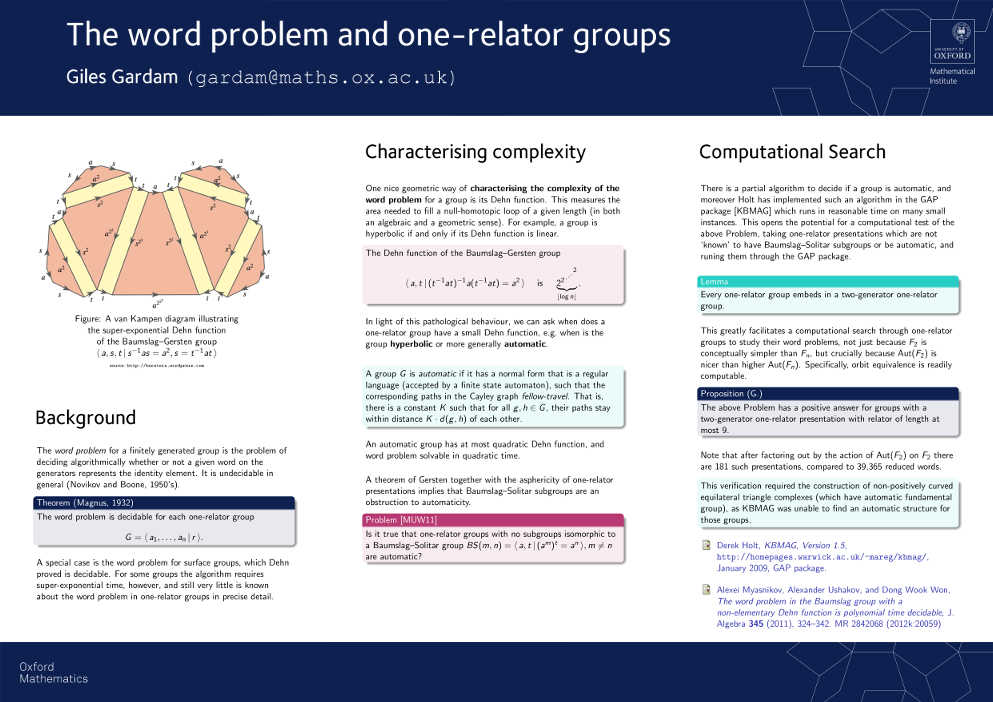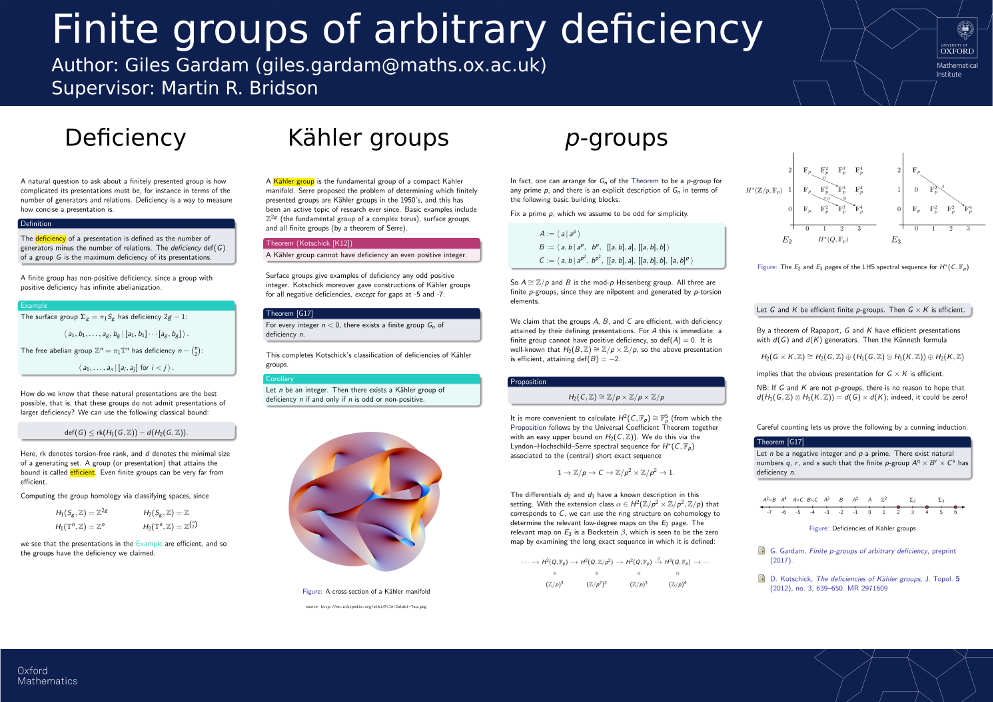## Where you might meet me

• No upcoming travel plans